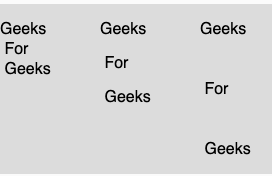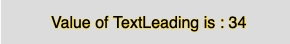The textAlign() function in p5.js is used to set the spacing, in pixels, between lines of text. This function is used in all subsequent calls to the text() function.

Syntax:

`textLeading(leading)`

Parameters: This function accepts single argument leading which stores the size in pixels for spacing between the lines.

Below programs illustrate the textLeading() function in p5.js:

Example 1: This example uses textAlign() function to set the spacing, in pixels, between lines of text.

 `function` `setup() { ` ` `  `    ``// Create Canvas of given size ` `    ``createCanvas(380, 170); ` `} ` ` `  `function` `draw() { ` `    ``let string = ``"Geeks \n For \n Geeks"``; ` `     `  `    ``// Set the background color ` `    ``background(220); ` `     `  `    ``// Set the text size ` `    ``textSize(16); ` `     `  `    ``// Set the text  ` `    ``text(string, 0, 30); ` `     `  `    ``// set the text leading ` `    ``textLeading(34) ` `     `  `    ``text(string, 100, 30); ` `     `  `    ``// set the text leading ` `    ``textLeading(60) ` `     `  `    ``text(string, 200, 30); ` `} `

Output:Example 2: This example uses textAlign() function to return the spacing, in pixels, between lines of text.

 `function` `setup() { ` ` `  `    ``// Create Canvas of given size ` `    ``createCanvas(380, 170); ` `} ` ` `  `function` `draw() { ` `     `  `    ``let string = ``"Geeks \n For \n Geeks"``; ` `     `  `    ``// Set the background color ` `    ``background(220); ` `     `  `    ``// Set the text size ` `    ``textSize(16); ` `     `  `    ``// Set the text leading ` `    ``textLeading(34) ` `     `  `    ``// Get the value of text leading ` `    ``var` `u = textLeading(); ` `     `  `    ``// Set the stroke color ` `    ``stroke(255, 204, 0); ` `     `  `    ``// Display the value ` `    ``text(``"Value of TextLeading is : "` `+ u, 50, 30); ` `} `

Output:Whether you're preparing for your first job interview or aiming to upskill in this ever-evolving tech landscape, GeeksforGeeks Courses are your key to success. We provide top-quality content at affordable prices, all geared towards accelerating your growth in a time-bound manner. Join the millions we've already empowered, and we're here to do the same for you. Don't miss out - check it out now!

Previous
Next